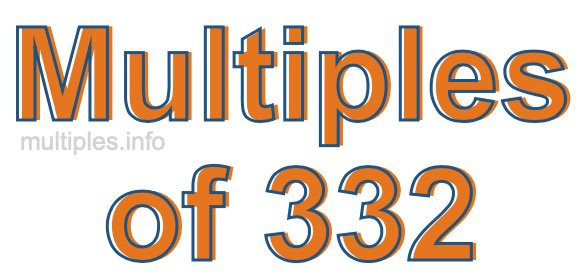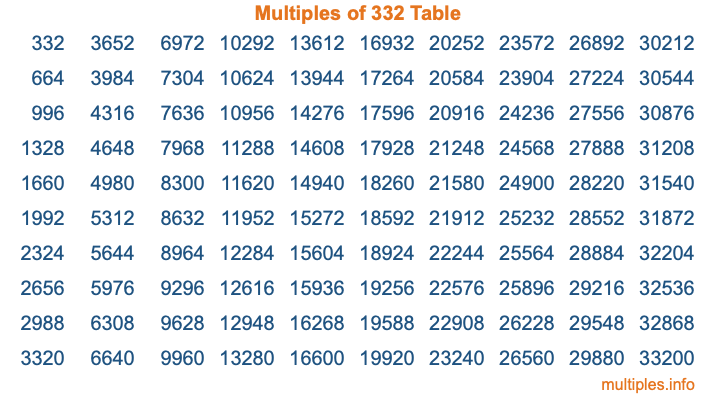Multiples of 332Welcome to the Multiples of 332 page. Here we will first teach you everything you will ever need to know about the multiples of 332, and then give you a study guide summary of everything we taught you to make sure you remember it all. Use this page to look up facts and learn information about the multiples of 332. This page will make you a multiples of three hundred thirty-two expert!

Definition of Multiples of 332
Multiples of 332 are all the numbers that when divided by 332 equal an integer. Each of the multiples of 332 are called a multiple. A multiple of 332 is created by multiplying 332 by an integer.

Therefore, to create a list of multiples of 332, you start with 1 multiplied by 332, then 2 multiplied by 332, then 3 multiplied by 332, and so on for as long as you want. Thus, the list of the first five multiples of 332 is 332, 664, 996, 1328, and 1660. To see a larger list of multiples of 332, see the printable image of Multiples of 332 further down on this page. We also have a category where you can choose any nth multiple of 332.

Multiples of 332 Checker
The Multiples of 332 Checker below checks to see if any number of your choice is a multiple of 332. In other words, it checks to see if there is any number (integer) that when multiplied by 332 will equal your number. To do that, we divide your number by 332. If the the quotient is an integer, then your number is a multiple of 332.

Is  a multiple of 332?

Least Common Multiple of 332 and ...
A Least Common Multiple (LCM) is the lowest multiple that two or more numbers have in common. This is also called the smallest common multiple or lowest common multiple and is useful to know when you are adding our subtracting fractions. Enter one or more numbers below (332 is already entered) to find the LCM.

Check out our LCM Calculator if you need more details about the Least Common Multiple or if you need the LCM for different numbers for adding and subtraction fractions.

nth Multiple of 332
As we stated above, 332 is the first multiple of 332, 664 is the second multiple of 332, 996 is the third multiple of 332, and so on. Enter a number below to find the nth multiple of 332.

th multiple of 332

Multiples of 332 vs Factors of 332
332 is a multiple of 332 and a factor of 332, but that is where the similarities end. All postive multiples of 332 are 332 or greater than 332. All positive factors of 332 are 332 or less than 332.

Below is the beginning list of multiples of 332 and the factors of 332 so you can compare:

Multiples of 332: 332, 664, 996, 1328, 1660, etc.

Factors of 332: 1, 2, 4, 83, 166, 332

As you can see, the multiples of 332 are all the numbers that you can divide by 332 to get a whole number. The factors of 332, on the other hand, are all the whole numbers that you can multiply by another whole number to get 332.

It's also interesting to note that if a number (x) is a factor of 332, then 332 will also be a multiple of that number (x).

Multiples of 332 vs Divisors of 332
The divisors of 332 are all the integers that 332 can be divided by evenly. Below is a list of the divisors of 332.

Divisors of 332: 1, 2, 4, 83, 166, 332

The interesting thing to note here is that if you take any multiple of 332 and divide it by a divisor of 332, you will see that the quotient is an integer.

Multiples of 332 Table
Below is an image of the first 100 multiples of 332 in a table. The table is in chronological order, column by column. The first column has the first ten multiples of 332, the second column has the next ten multiples of 332, and so on.The Multiples of 332 Table is also referred to as the 332 Times Table or Times Table of 332. You are welcome to print out our table for your studies.

Negative Multiples of 332
Although not often discussed or needed in math, it is worth mentioning that you can make a list of negative multiples of 332 by multiplying 332 by -1, then by -2, then by -3, and so on, to get the following list of negative multiples of 332:

-332, -664, -996, -1328, -1660, etc.

Multiples of 332 Summary
Below is a summary of important Multiples of 332 facts that we have discussed on this page. To retain the knowledge on this page, we recommend that you read through the summary and explain to yourself or a study partner why they hold true.

There are an infinite number of multiples of 332.

A multiple of 332 divided by 332 will equal a whole number.

332 divided by a factor of 332 equals a divisor of 332.

The nth multiple of 332 is n times 332.

The largest factor of 332 is equal to the first positive multiple of 332.

332 is a multiple of every factor of 332.

332 is a multiple of 332.

A multiple of 332 divided by a divisor of 332 equals an integer.

332 divided by a divisor of 332 equals a factor of 332.

Any integer times 332 will equal a multiple of 332.

Multiples of a Number
Here you can get the multiples of another number, all with the same attention to detail as we did for multiples of 332 on this page.

Multiples of
Multiples of 333
Did you find our page about multiples of three hundred thirty-two educational? Do you want more knowledge? Check out the multiples of the next number on our list!